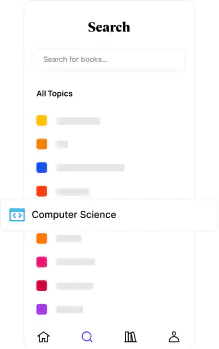📖[PDF] Matrices and Linear Transformations by Charles G. Cullen | Perlego
Start your free trial today and explore our endless library.📖 Book - PDF

# Matrices and Linear Transformations

Second Edition
Charles G. CullenShare book336 pagesEnglishePUB (mobile friendly) and PDFAvailable on iOS & Android
📖 Book - PDF

# Matrices and Linear Transformations

Second Edition
Charles G. Cullen
Book details
Citations

`Comprehensive . . . an excellent introduction to the subject.` — Electronic Engineer's Design Magazine.
This introductory textbook, aimed at sophomore- and junior-level undergraduates in mathematics, engineering, and the physical sciences, offers a smooth, in-depth treatment of linear algebra and matrix theory. The major objects of study are matrices over an arbitrary field.
Contents include Matrices and Linear Systems; Vector Spaces; Determinants; Linear Transformations; Similarity: Part I and Part II; Polynomials and Polynomial Matrices; Matrix Analysis; and Numerical Methods.
The first seven chapters, which require only a first course in calculus and analytic geometry, deal with matrices and linear systems, vector spaces, determinants, linear transformations, similarity, polynomials, and polynomial matrices. Chapters 8 and 9, parts of which require the student to have completed the normal course sequence in calculus and differential equations, provide introductions to matrix analysis and numerical linear algebra, respectively. Among the key features are coverage of spectral decomposition, the Jordan canonical form, the solution of the matrix equation AX = XB, and over 375 problems, many with answers.

## Information

Publisher
Dover Publications
Year
2012
ISBN
9780486132419
Topic
Mathematics
Subtopic
Algebra
Edition
2